# Know Your 2D Shapes: What are They Called?

In this worksheet, students recognise a 2D shape and select its name.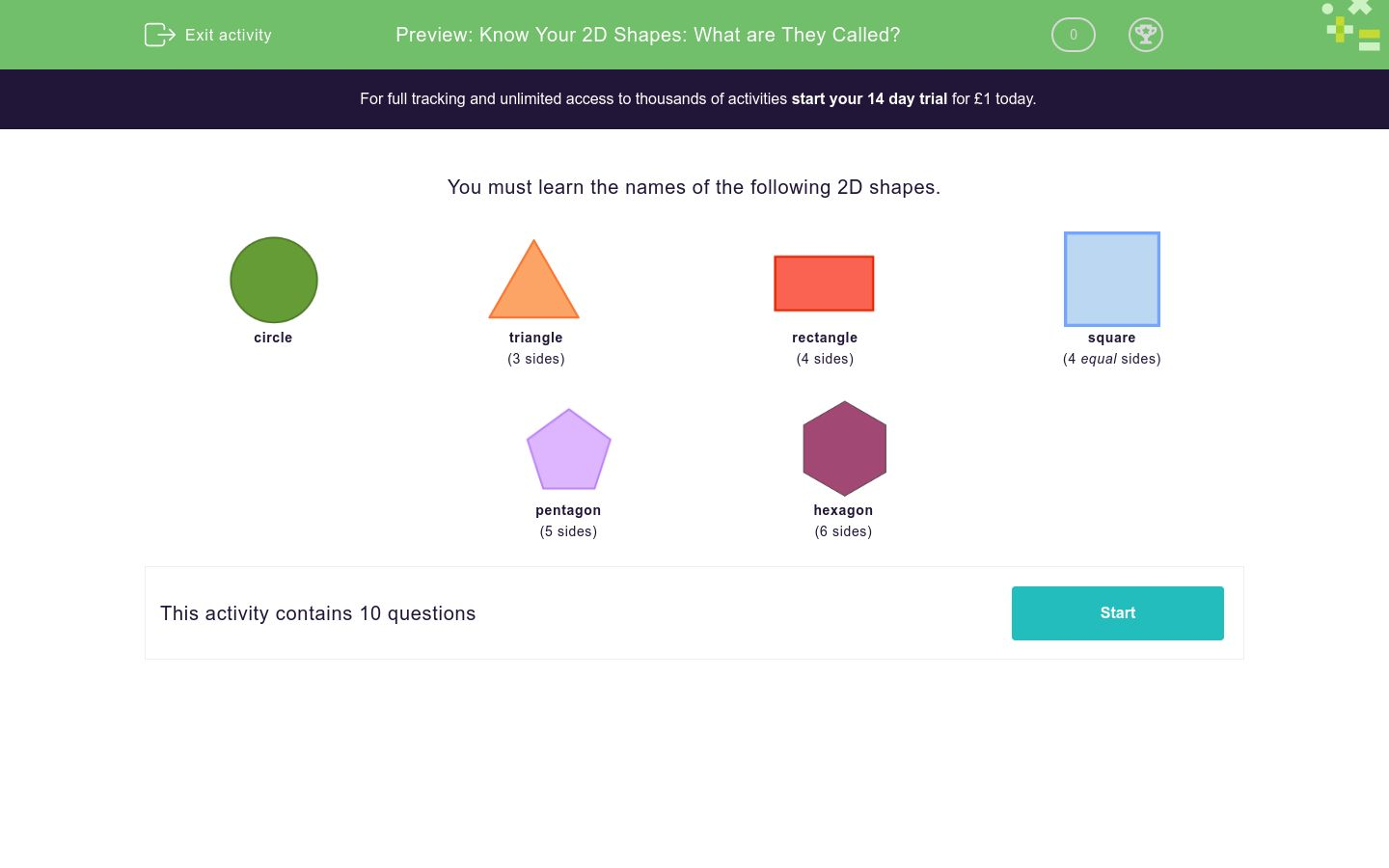Key stage:  KS 1

Curriculum topic:   Geometry: Properties of Shapes

Curriculum subtopic:   Compare 2D and 3D Shapes

Difficulty level:### QUESTION 1 of 10

You must learn the names of the following 2D shapes.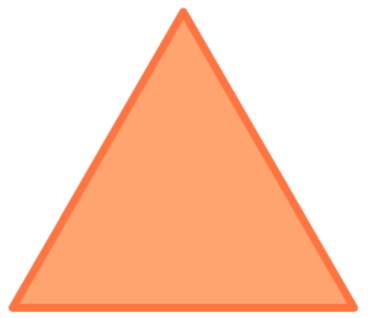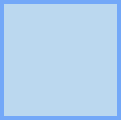circle triangle (3 sides) rectangle (4 sides) square (4 equal sides)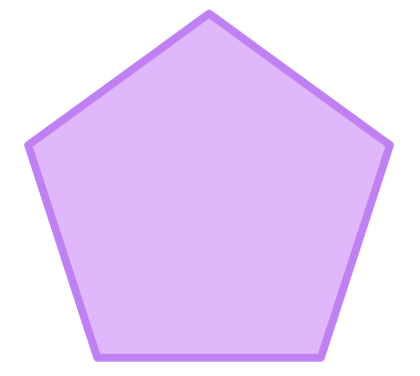pentagon (5 sides) hexagon (6 sides)

What is this shape called?hexagon

pentagon

rectangle

square

triangle

circle

What is this shape called?hexagon

pentagon

rectangle

square

triangle

circle

What is this shape called?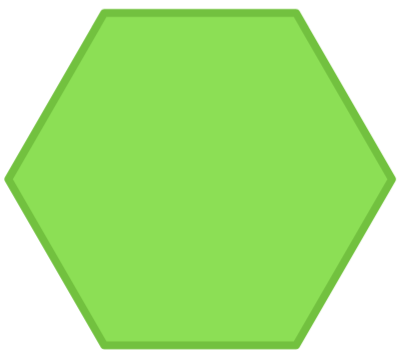hexagon

pentagon

rectangle

square

triangle

circle

What is this shape called?hexagon

pentagon

rectangle

square

triangle

circle

What is this shape called?hexagon

pentagon

rectangle

square

triangle

circle

What is this shape called?hexagon

pentagon

rectangle

square

triangle

circle

What is this shape called?hexagon

pentagon

rectangle

square

triangle

circle

What is this shape called?hexagon

pentagon

rectangle

square

triangle

circle

What is this shape called?hexagon

pentagon

rectangle

square

triangle

circle

What is this shape called?hexagon

pentagon

rectangle

square

triangle

circle

• Question 1

What is this shape called?circle
EDDIE SAYS
It is curved.
• Question 2

What is this shape called?square
EDDIE SAYS
It has 4 equal sides.
• Question 3

What is this shape called?hexagon
EDDIE SAYS
It has 6 sides.
• Question 4

What is this shape called?rectangle
EDDIE SAYS
It has 4 sides and 4 right angles. Opposite sides are equal.
• Question 5

What is this shape called?triangle
EDDIE SAYS
It has 3 sides.
• Question 6

What is this shape called?pentagon
EDDIE SAYS
It has 5 sides.
• Question 7

What is this shape called?rectangle
EDDIE SAYS
It has 4 sides and 4 right angles. Opposite sides are equal.
• Question 8

What is this shape called?square
EDDIE SAYS
It has 4 equal sides.
• Question 9

What is this shape called?rectangle
EDDIE SAYS
It has 4 sides and 4 right angles.
• Question 10

What is this shape called?circle
EDDIE SAYS
It is curved.
---- OR ----

Sign up for a £1 trial so you can track and measure your child's progress on this activity.

### What is EdPlace?

We're your National Curriculum aligned online education content provider helping each child succeed in English, maths and science from year 1 to GCSE. With an EdPlace account you’ll be able to track and measure progress, helping each child achieve their best. We build confidence and attainment by personalising each child’s learning at a level that suits them.

Get started